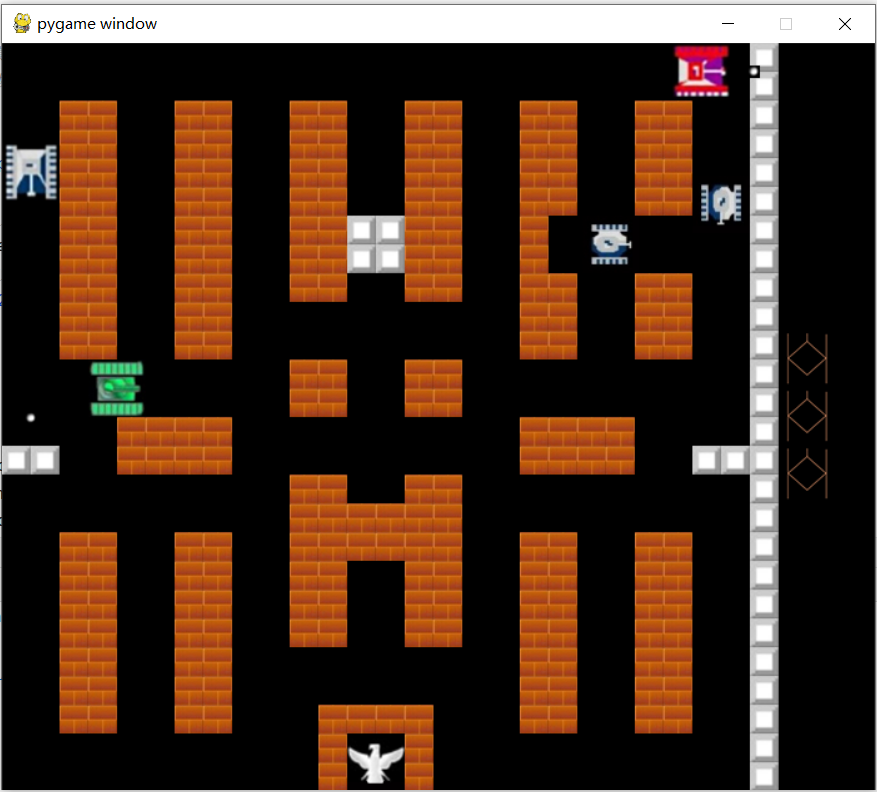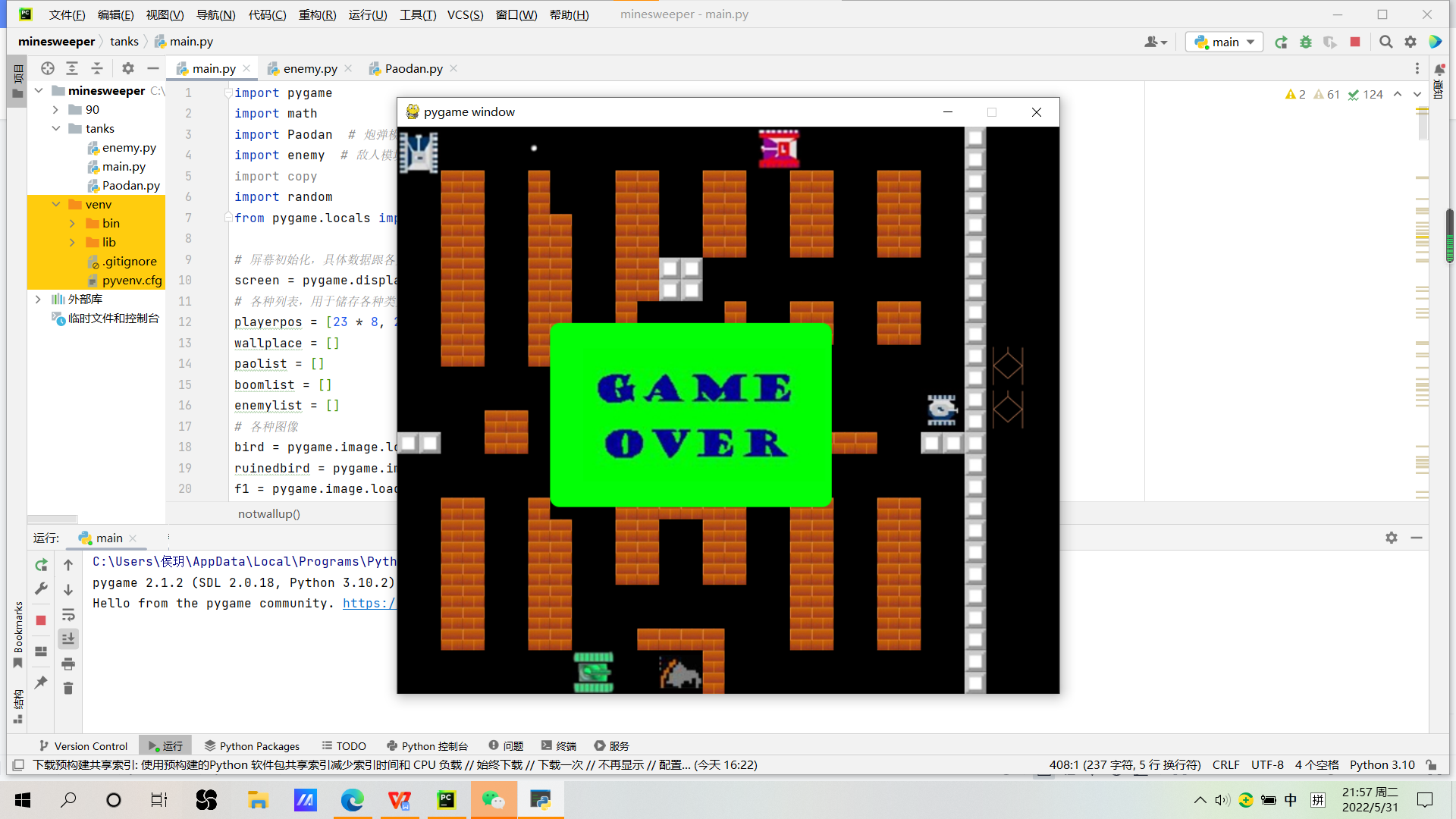# 20212102侯玥实验四pygame之坦克大战

PART1：坦克大战代码分析

1. 游戏元素共分bullets，tanks，walls，booms，bird，enemies六种。
2. 对于tanks类：由于各游戏元素的导入为图像形式，因此要实现元素的动态效果需要不断刷新屏幕。动态效果涉及一组两张图片加载，通过mf%2来选择状态。

event.key == K_s and playerpos % 23 == 0 and playerpos % 23 == 0
1. 对于bullets，也就是炮弹类（因为英文有点长在程序里就写的pao）：

class Pao(object):
def __init__(self,paopos,Direction,source):
self.paopos = paopos
self.Direction =Direction
self.source=source


1. 对于boom类：

Pao元素遇到只剩一滴血的坦克元素才会发生boom。

for i in paolist:
flag = False

if i.source == 0:
for j in enemylist:
if -23 < i.paopos - j.enemypos <= 46 and -23 < i.paopos - j.enemypos <= 46:
if (j.blood == 1):
enemylist.remove(j)
enemynum -= 1
else:
j.blood -= 1
flag = True
break


Pao元素遇到特殊墙体后也会发生boom（以向上走的炮弹为例）。

if i.Direction == 0:
if [[i.paopos // 23, math.ceil(i.paopos / 23) - 1], 0] in wallplace:
flag = True
wallplace.remove([[i.paopos // 23, math.ceil(i.paopos / 23) - 1], 0])
if [[i.paopos // 23 + 1, math.ceil(i.paopos / 23) - 1], 0] in wallplace:
flag = True
wallplace.remove([[i.paopos // 23 + 1, math.ceil(i.paopos / 23) - 1], 0])

#0类墙体表示木质墙体，被炮弹攻击会消失半格
if [[i.paopos // 23, math.ceil(i.paopos / 23) - 1], 1] in wallplace or [
[i.paopos // 23, math.ceil(i.paopos / 23) - 1], 2] in wallplace:
flag = True
if [[i.paopos // 23 + 1, math.ceil(i.paopos / 23) - 1], 1] in wallplace or [
[i.paopos // 23 + 1, math.ceil(i.paopos / 23) - 1], 2] in wallplace:
flag = True
#1类或2类墙体表示特殊墙体，无法被摧毁
if flag:
boomlist.append([[i.paopos - 9, i.paopos - 14], 5])
paolist.remove(i)


Pao遇到pao也会boom。

1. 对于enemy类：
def __init__(self,enemypos,Direction,Type,blood,speed,frequency):


def enemyattack():
for i in enemylist:
if i.frequency != 0:
i.frequency -= 1
if i.enemypos % 23 == 0 and i.enemypos % 23 == 0 and i.frequency == 0:
if random.randint(0, 1) == 0:
paolist.append(Paodan.Pao(setpao(i.Direction, i.enemypos), i.Direction, 1))
i.frequency = 150


for i in enemylist:
point = False
flag = False
if i.Direction == 0 and notwallup(i.enemypos, 0) and notwallup(i.enemypos, 1) and notwallup(i.enemypos,
2) and notwallup(
i.enemypos, 4):
for j in enemylist:
if i.enemypos != j.enemypos and 0 < i.enemypos - j.enemypos <= 46 and abs(
j.enemypos - i.enemypos) <= 46:
point = True
if point != True:
i.enemypos -= i.speed
flag = True


1. 对于wall类：

Pao遇到木质墙体会将墙体移除：

if [[i.paopos // 23 + 1, math.ceil(i.paopos / 23) - 1], 0] in wallplace:
flag = True
wallplace.remove([[i.paopos // 23 + 1, math.ceil(i.paopos / 23) - 1], 0])


def notwallup(pos, Type):
if [[pos // 23, math.ceil(pos / 23) - 1], Type] not in wallplace and [
[pos // 23 + 1, math.ceil(pos / 23) - 1], Type] not in wallplace:
return True
else:
return False


（这里插一句，游戏中涉及到敌我坦克不可穿模问题，所以将所有坦克按特殊墙体处理）

1. 各元素坐标初始化：
playerpos = [23 * 8, 23 * 24] #坦克坐标初始化
wallplace = []
paolist = []
boomlist = []
enemylist = []


1. 调用
pygame.image.load('').convert_alpha()


1. 调用
pygame.time.delay(1)


1. Enemy出生点设置：
if rspawnpoint == mspawnpoint == lspawnpoint == 0 and enemynum != 4:

#敌人出生点设置，保证界面中有不少于4名敌人
enemynum += 1
if random.randint(0, 2) == 0:
rspawnpoint = 600
elif random.randint(0, 1) == 0:
mspawnpoint = 600
else:
lspawnpoint = 600
#随机选择出生点
else:
pass


1. 无墙正常行走时（以向左为例）：
def notwallleft(pos, Type):
if [[math.ceil(pos / 23) - 1, pos // 23], Type] not in wallplace and [
[math.ceil(pos / 23) - 1, pos // 23 + 1], Type] not in wallplace:
return True
else:
return False


PART2：结果如下PART3：感想

90tank这部游戏可以说有我童年的回忆在，我和我表哥小时候经常在一起打。听说可以做游戏于是立马选择了这个。但后来发现游戏程序的编写不是易事。

Python实现的是2D游戏，画面元素通过图片形式加载，所以想要做出游戏的动态效果是很麻烦的。每个坦克有两种状态，用于实现图片的动态效果，但代码也就会变得很长，于是我的图片加载代码差不多快100行了......

posted @ 2022-05-31 23:22  FayFloride  阅读(47)  评论(0编辑  收藏  举报